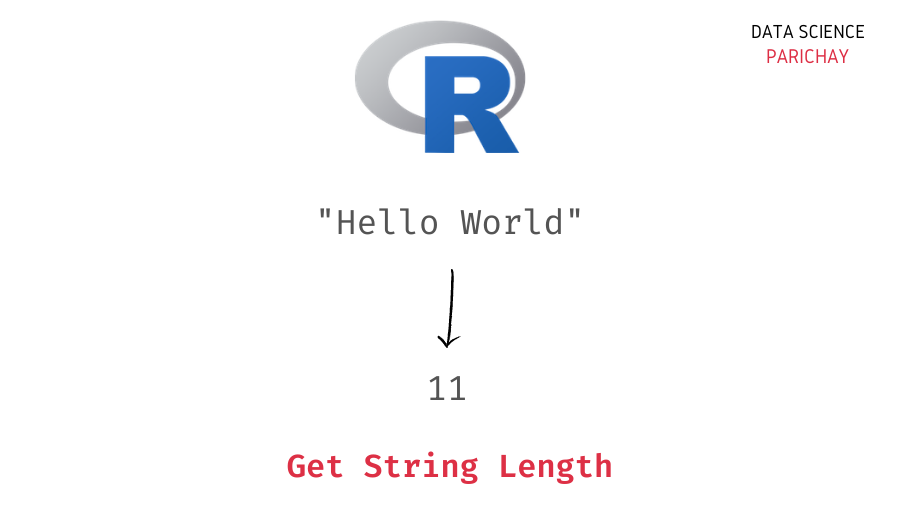# Find the Length of a String in R (With Examples)

The R programming language comes with a number of built-in functions to work with character strings. In this tutorial, we will look at one such function that helps us get the length of a string in R with the help of some examples.

## How to find the length of a string in R?

You can use the built-in `nchar()` function to get the length of a string in R. Pass the string as an argument to the function.

The following is the syntax –

```# length of string s
nchar(s)```

The `nchar()` function returns the number of characters in the string (which is the same as the length of the string).

## Examples

Let’s now look at some examples of using the above syntax.

### Length of a string using `nchar()`

Let’s apply the `nchar()` function on a string, for example, “Avengers Endgame”.

```# create a string
s <- "Avengers Endgame"
# get length of s
print(nchar(s))```

Output:

` 16`

We get 16 as the length of the string “Avengers Endgame”. This is the correct length since there is a total of 16 characters in the above string.

📚 Data Science Programs By Skill Level

Introductory

Intermediate ⭐⭐⭐

🔎 Find Data Science Programs 👨‍💻 111,889 already enrolled

Disclaimer: Data Science Parichay is reader supported. When you purchase a course through a link on this site, we may earn a small commission at no additional cost to you. Earned commissions help support this website and its team of writers.

### Length of each string in a vector

If you apply the `nchar()` function to a string vector, it will return a vector with the length of each corresponding string in the vector.

Let’s look at an example.

```# create a vector of strings
vec <- c("you", "are", "my", "fire")
# get length of each string in vec
print(nchar(vec))```

Output:

` 3 3 2 4`

You can see that we get the length of each string the vector `vec` with the `nchar()` function.

What if the string vector has `NA` values?

Let’s find out.

This time, we’ll add some `NA` values to the above vector and then apply the `nchar()` function.

```# create a vector of strings with some NA values
vec <- c("you", "are", NA, "my", "fire", NA)
# get length of each string in vec
print(nchar(vec))```

Output:

`  3  3 NA  2  4 NA`

We get the length for each valid string and `NA` for the `NA`s in the above vector.

You might also be interested in –

•